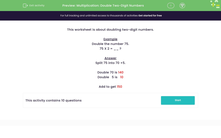# Double Two-Digit Numbers Using Partitioning

In this worksheet, students will use a strategy that helps them to double (or multiply by 2) two-digit numbers.Key stage:  KS 2

Curriculum topic:   Number: Multiplication and Division

Curriculum subtopic:   Solve Multiplication Problems

Difficulty level:#### Worksheet Overview

This activity is about doubling two-digit numbers.

Doubling means multiplying a number by 2.

Example

Double the number 75.

75 x 2 = __ ?

Split 75 into 70 + 5.

Double 70 is 140

Double   5 is   10

Add 140 + 10  to get 150

How does that sound - easy, yes?

Let's try some questions now to practise this skill.### What is EdPlace?

We're your National Curriculum aligned online education content provider helping each child succeed in English, maths and science from year 1 to GCSE. With an EdPlace account you’ll be able to track and measure progress, helping each child achieve their best. We build confidence and attainment by personalising each child’s learning at a level that suits them.

Get started